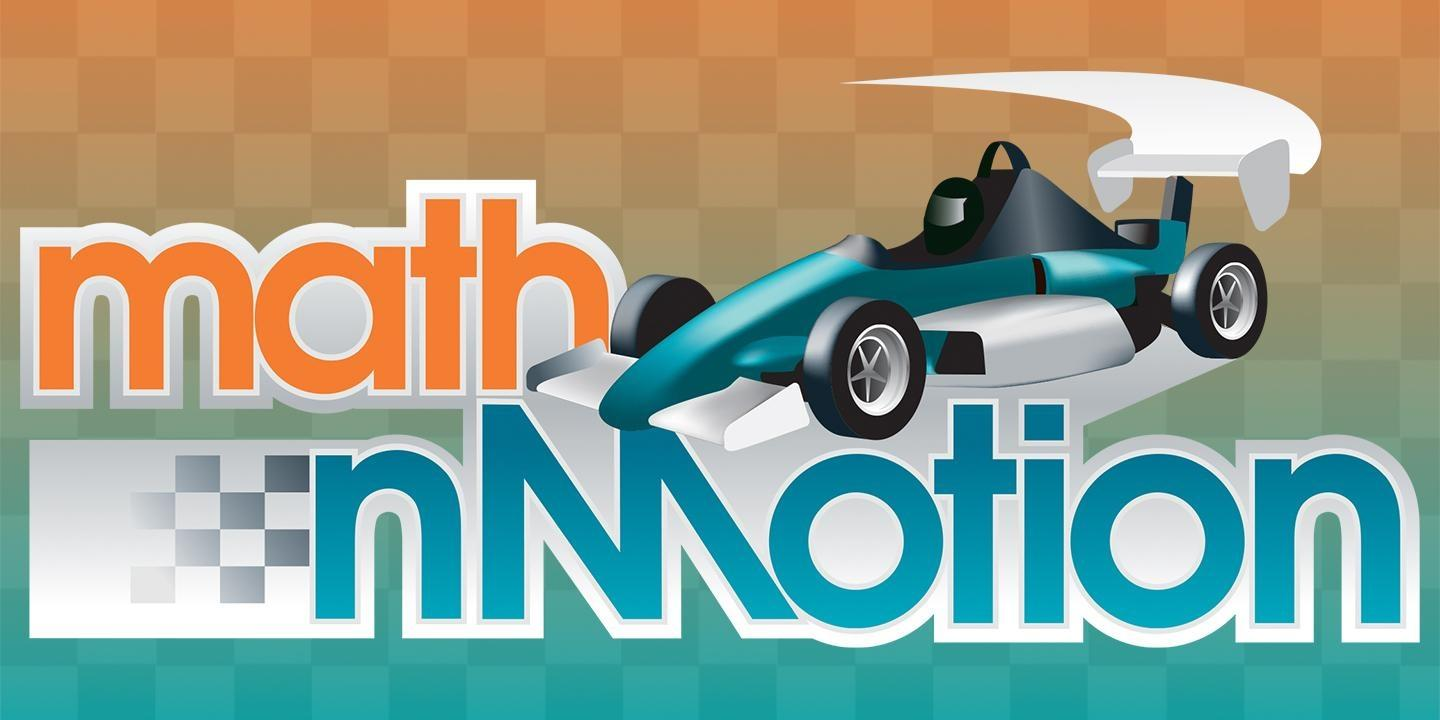# Math nMotion

MATH nMOTION tackles the very difficult concepts of graphing linear equations, inequalities, ratio and proportion and using data to make decisions through the example of racing Formula M cars.

It is keyed to 8th grade mathematics standards. Each section of this multimedia package contains the following components:

• a three- to four-minute video in .m4v format that gives a brief overview of the concept and real-life examples of why we need to know it
• a set of lesson plans that teach the concept
• a professional development video that explains the main focus of the segment and what is included in each lesson
• a formative and summative assessment for each section

Also included are resource sheets on the Formula M car, the TI-84 graphing calculator and on using Excel.

# Educational Resources

Produced: 2009

Subject Area: Mathematics

Resources: Math nMotion Teacher Guide

## Student Videos

### Ratio and Proportion

Understanding ratio, proportion and percent is a critical skill that is important when solving real-life problems. It is also a stepping stone to understanding more complicated mathematical concepts.

### Graphing Linear Equations

The coordinate plane is a two-dimensional surface on which we can plot points, lines and curves. It has two scales, called the x-axis and y-axis, at right angles to each other that meet at the point of origin (0, 0). Students are given real-life examples of how and why they could create and use a line to give them information that will help them to solve problems.

### Inequalities

In this module, students use the concept of Formula M racing to understand the concept of inequalities. What does it mean if a solid line is found on the plane and all areas below the line are shaded? This could mean that anything on the line is a tie in the race and anything in the shaded area would be a loss. In other words, we're looking at real-life activities to teach this abstract concept.

### Using Data to Make Decisions

We are inundated with information—about our health, our leisure activities, sports and so much more. Information is just information. It is our responsibility to process that information and to use it wisely. We need to not only evaluate this information, but also to base personal decisions on evidence (data). This module shows us how race car drivers use data to help them win races.